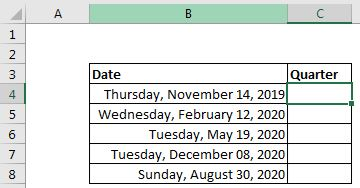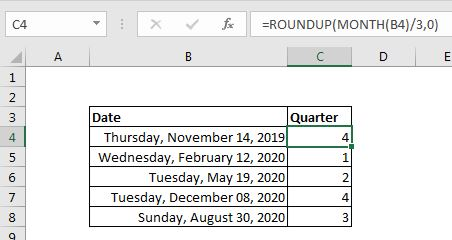# How to Calculate Quarter in Excel

Sometimes, we need to calculate the Quarter of the years of a specified date. For example, if the quarter of 1-jan-2020 is 1, the quarter of 2-Jul-2019 is 3, etc.

In this article, we will simply learn how can you easily calculate the quarter of a given date using a simple quarter formula in Excel.

The generic formula for calculating Quarter of the year of a given date is:

 =ROUNDUP(MONTH(date)/3,0)

Date: The date is a valid Excel date. It can be a cell a reference to a date, a calculated date or a hardcoded date.

Let's see this formula in action.

### Example: Calculate the year quarter of the given dates:

Here, we have some dates in range  B2:B8. We need to calculate the quarter they fall in from the four quarters of the year.Using the above generic formula, we can write this formula in cell C4 to get the quarter of the first date and then copy the formula down to get the quarter of each date in the relative left cell.

 =ROUNDUP(MONTH(B4)/3,0)The above function returns the quarter of the first date Thursday, November 14, 2019, as 4 as the November month is 4th quarter of the year.

How does it work?

As we know that a year has four quarters. Q1 has Jan, Feb, and Mar. Q2 has Apr, May, and June. Q3 covers Jul, Aug, and Sep and Q4 has Oct, Nov, and Dec. If we divide the month number by 3 then the number we will get will always be less than or equal to the quarter of that month number and greater than quarter before it. I may have complicated here. Let's see the explanation.

The formula is simple. As all the function starts solving from inside, let's start from the center.

First, we use the Excel Function MONTH. The MONTH function returns the month in which that date falls. The first date falls in 11th months hence it returns 11.

Next, we divide the month number from 3. This returns us a fractional number that is 3.666667.

Finally, we encapsulate this number with the ROUNDUP Function to the 0 decimal numbers. This returns the whole number 4. Which is the quarter of the first date?

So yeah guys, this how you can get the year quarter of date in Excel using one single formula. I have tried to be explanatory enough. If you have any doubts regarding this formula or have any other excel VBA related query, ask in the comments section below.

Related Articles:

How to Determine Fiscal Quarter of Date in Excel |  Fiscal Quarter of year is 1/3 part of the financial year of a company. Suppose a company yearly period is Feb'19-Jan'20, then the first fiscal quarter of the company's year will contain month's Feb'19, Mar'19, and Apr'19.

Conditional formatting based on another cell value: To format cells based on the value of other cells, we just set formula on another cell while having selected the formatting cells.

IF and Conditional formattingThe IF and the condition are so interrelated. The If can also be used to conditionally format cells.

Conditional Formatting with formula 2016The conditional formatting can be done with formulas too. To use formulas for determining the cells that you want to format.

Highlight cells that contain specific textTo highlight cells that contain a specific text, simply use the predefined rule of  conditional formatting.

Popular Articles:

50 Excel Shortcuts to Increase Your Productivity | Get faster at your task. These 50 shortcuts will make you work even faster on Excel.

The VLOOKUP Function in Excel | This is one of the most used and popular functions of excel that is used to lookup value from different ranges and sheets.

COUNTIF in Excel 2016 | Count values with conditions using this amazing function. You don't need filter your data to count specific value. Countif function is essential to prepare your dashboard.

How to Use SUMIF Function in Excel | This is another dashboard essential function. This helps you sum up values on specific conditions.

Terms and Conditions of use

The applications/code on this site are distributed as is and without warranties or liability. In no event shall the owner of the copyrights, or the authors of the applications/code be liable for any loss of profit, any problems or any damage resulting from the use or evaluation of the applications/code.